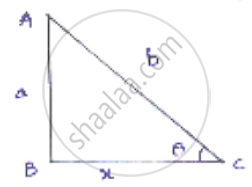Share

# If Sin Theta = A/B Find Sec θ + Tan θ in Terms of a and B. - CBSE Class 10 - Mathematics

#### Question

If sin theta = a/b find sec θ + tan θ in terms of a and b.

#### Solution

We know sin theta = "𝑜𝑝𝑝𝑜𝑠𝑖𝑡𝑒 𝑠𝑖𝑑𝑒"/"ℎ𝑦𝑝𝑜𝑡𝑒𝑛𝑢𝑠𝑒"Let x be the adjacent side

By applying Pythagoras theorem

𝐴𝐶2 = 𝐴𝐵2 + 𝐵𝐶2

b2 = a2 + x2

x2 = b2 − a2

x = sqrt(b^2 - a^2)

sec theta = (AB)/(BC) = b/(sqrt(b^2 - a^2))

tan theta = (AB)/(BC) = a/(sqrt(b^2 - a^2))

sec theta + tan theta = b/(b^2 - a^2) + a/(sqrt(b^2 - a^2))

= (b + a)/(sqrt(b^2 - a^2)) = (b+ a)/sqrt((b + a)(b - a)) = (b + a)/sqrt(b + a) - 1/(sqrt(b - a)) = sqrt((b + a)/(b - a))

Is there an error in this question or solution?

#### APPEARS IN

Solution If Sin Theta = A/B Find Sec θ + Tan θ in Terms of a and B. Concept: Trigonometric Ratios.
S